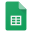# Elementary Combinatorics & ProbabilityBarcelona

## Lectures

Lecture 1 • Sets, Multiplication and Addition Principles - PDF - PDF

Lecture 2 • The Principle of Inclusion-Exclusion - PDF

Lecture 3 • Permutations and Combinations

Lecture 4 • Applications of Permutations and Combinations - PDF

Lecture 5 • The Pigeonhole Principle - PDF

Lecture 6 • The Probability of an Event - PDF

Lecture 7 • The Probability of an Event

Lecture 8 • Conditional Probability and Independent Events - PDF

Lecture 9Random Variables and Expected Values

Project • Modeling Blackjack - PDF

## Homework

Homework 1 • 14, 25, 28, 31, 33 - Sets, Multiplication and Addition Principles

Homework 2 • 4, 12, 14, 15, 17 - The Principle of Inclusion-Exclusion

Homework 3 • 34, 38, 44, 52, 54 - Permutations and Combinations

Homework 4 • 1, 3, 6, 7, 12 - Applications of Permutations and Combinations

Homework 5 • 4, 6, 12, 15, 20 - The Pigeonhole Principle

Homework 6 • 9, 12, 17, 28, 39 - The Probability of an Event

Homework 7 • 49, 61, 67, 72, 73 - The Probability of an Event

Homework 8 • 3, 7, 8, 19, 20 - PDF (Section 10.2)

Homework 9 • 2, 4, 6, 8, 10 - PDF (Section 10.3)

## ResultsResults / Elementary Combinatorics & Probability

* The percentage of your homework is calculated as the maximum percentage times the ratio of your score to the maximum score

** In-class participation takes into account your class attendance and involvement in the process

## Literature

1. Discrete Mathematics, Gary Chartrand, Ping Zhang, Waveland Press 2011

2. Introduction to Counting & Probability, David Patrick, The Art of Problem Solving 2007

3. Schaum's Outline of Probability and Statistics, John Schiller, R. Alu Srinivasan, Murray Spiegel, McGraw-Hill 2008

4. Precalculus, Michael Sullivan, Pearson 2017

5. Handbook of Discrete and Combinatorial Mathematics, Kenneth H. Rosen (Editor), CRC Press 2000